O 1 O O 1 O O 1 O 1 1 O 1 1 O 1 O 1 1 O O O O 1 O 1 1 O O 1 1 1 O 1 1 O 1 O O 1 O 1 1 O 1 1 1 O O 1 1 O O O O 1 O 1 1 1 O 1 O O O 1 1 O 1 O O 1 O 1 1 O 1 1 1 1 O 1 1 O 1 1 1 O O O 1 O O O O O O 1 1 O 1 O O 1 O 1 1 1 O O 1 1 O O 1 O O O O O O 1 1 O 1 1 O 1 O 1 1 O 1 1 1 1 O 1 1 1 O O 1 O O 1 1 O O 1 O 1 O O 1 O O O O O O 1 1 O 1 O O 1 O 1 1 O 1 1 O 1 O 1 1 1 O O O O O 1 1 O 1 1 1 1 O 1 1 1 O O 1 O O 1 1 1 O 1 O O O 1 1 O O O O 1 O 1 1 O 1 1 1 O O 1 1 1 O 1 O O O O 1 O O O O O O 1 1 1 O 1 O O O 1 1 O 1 O O O O 1 1 O O O O 1 O 1 1 O 1 1 1 O O O 1 O O O O O O 1 1 O 1 O 1 1 O 1 1 O 1 1 1 O O 1 1 O 1 1 1 1 O 1 1 1 O 1 1 1 O 1 1 O 1 1 O O O 1 1 O O 1 O 1 O 1 1 O O 1 O O O 1 1 O O 1 1 1 O 1 1 O O 1 O 1 O O 1 O 1 1 1 O O O 1 O O O O O O 1 O O O 1 1 O O 1 1 O 1 1 1 1 O 1 1 1 O O 1 O O O 1 O O O O O O 1 1 O 1 O 1 1 O 1 1 O 1 1 1 O O 1 1 O 1 1 1 1 O 1 1 1 O 1 1 1 O 1 1 O 1 1 O O O 1 1 O O 1 O 1 O 1 1 O O 1 O O O 1 1 O O 1 1 1 O 1 1 O O 1 O 1 O O 1 O O O O O O 1 1 O 1 O O 1 O 1 1 1 O O 1 1 O O 1 O O O O O O 1 1 O 1 1 O O O 1 1 O 1 O O 1 O 1 1 O 1 1 O 1 O 1 1 O 1 O O 1 O 1 1 1 O 1 O O O 1 1 O O 1 O 1 O 1 1 O O 1 O O O O 1 O O O O O O 1 1 1 O 1 O O O 1 1 O 1 1 1 1 O O 1 O O O O O O 1 1 O O O O 1 O 1 1 O 1 1 O O O 1 1 O 1 1 O O O O 1 O O O O O O 1 1 1 O 1 1 1 O 1 1 O O 1 O 1 O O 1 O O O O O O 1 1 O 1 1 1 O O 1 1 O 1 1 1 1 O 1 1 1 O 1 1 1 O O 1 O O O O O O 1 1 O 1 O 1 1 O 1 1 O 1 1 1 O O 1 1 O 1 1 1 1 O 1 1 1 O 1 1 1 O O 1 O O O O O O 1 1 O O O O 1 O 1 1 O 1 1 1 O O 1 1 O O 1 O O O O 1 O O O O O O 1 1 1 O 1 O 1 O 1 1 O 1 1 1 O O 1 1 O O 1 O O O 1 1 O O 1 O 1 O 1 1 1 O O 1 O O 1 1 1 O O 1 1 O 1 1 1 O 1 O O O 1 1 O O O O 1 O 1 1 O 1 1 1 O O 1 1 O O 1 O O O O 1 O 1 1 O O O O 1 O O O O O O 1 1 1 O 1 1 1 O 1 1 O 1 O O O O 1 1 O 1 O O 1 O 1 1 O 1 1 O O O 1 1 O O 1 O 1 O O 1 O O O O O O 1 1 O 1 O O 1 O 1 1 O 1 1 O 1 O 1 1 O O O O 1 O 1 1 O O 1 1 1 O 1 1 O 1 O O 1 O 1 1 O 1 1 1 O O 1 1 O O O O 1 O 1 1 1 O 1 O O O 1 1 O 1 O O 1 O 1 1 O 1 1 1 1 O 1 1 O 1 1 1 O O O 1 O O O O O O 1 1 O O 1 O 1 O 1 1 O 1 1 O 1 O 1 1 O O O 1 O O 1 1 1 O O 1 O O 1 1 O O O O 1 O 1 1 O O O 1 1 O 1 1 O O 1 O 1 O 1 1 1 O O 1 1 O O 1 O O O O O O 1 1 1 O 1 O O O 1 1 O 1 O O O O 1 1 O O 1 O 1 O O 1 O O O O O O 1 1 O O 1 O 1 O 1 1 O 1 1 1 O O 1 1 1 O 1 O O O 1 1 O 1 O O 1 O 1 1 1 O O 1 O O 1 1 O O 1 O 1 O O 1 O O O O O O 1 1 1 O 1 1 1 O 1 1 O 1 1 1 1 O 1 1 1 O O 1 O O 1 1 O 1 1 O O O 1 1 O O 1 O O O O 1 O 1 1 O O O O 1 O O O O O O 1 1 O O O O 1 O 1 1 O 1 1 1 O O 1 1 O O 1 O O O O 1 O O O O O O 1 1 O O O O 1 O 1 1 O 1 1 O O O 1 1 O 1 1 O O O O 1 O O O O O O 1 1 1 O 1 O O O 1 1 O 1 O O O O 1 1 O O 1 O 1 O 1 1 1 O O 1 O O 1 1 O O 1 O 1 O O 1 O O O O O O 1 1 O O 1 O 1 O 1 1 1 O 1 1 O O 1 1 O O 1 O 1 O 1 1 1 O O 1 O O O 1 O O O O O O 1 1 1 O 1 1 1 O 1 1 O 1 O O 1 O 1 1 O 1 1 O O O 1 1 O 1 1 O O O O 1 O O O O O O 1 1 O O O 1 O O 1 1 O O 1 O 1 O O 1 O O O O O O 1 1 1 O 1 O O O 1 1 O 1 1 1 1 O O 1 O O O O O O 1 1 O 1 O 1 1 O 1 1 O 1 1 1 O O 1 1 O 1 1 1 1 O 1 1 1 O 1 1 1 O O 1 O O O O O O 1 1 O O O O 1 O 1 1 O 1 1 1 O O 1 1 O O 1 O O O O 1 O O O O O O 1 1 1 O 1 O 1 O 1 1 O 1 1 1 O O 1 1 O O 1 O O O 1 1 O O 1 O 1 O 1 1 1 O O 1 O O 1 1 1 O O 1 1 O 1 1 1 O 1 O O O 1 1 O O O O 1 O 1 1 O 1 1 1 O O 1 1 O O 1 O O
Subliminal Video# Presentation

Subliminal Video is an application that allows you to add hidden images and messages in your videos.
Select and customize as many video frames as you want, replacing them with new images and texts.

Most of the viewers won't consciously see those subliminal images, which are displayed for a very short time.
They can, however, have an unconscious impact on their mind.
Use this application just for fun, or to trick your friends.

It is now possible to create videos like the famous “Drink Coca-Cola” and “Eat Popcorn” controversial subliminal advertising (in 1957 by James M. Vicary).
More recently, the movie Fight Club also used subliminal flashes to insert hidden pictures of Tyler Durden at different points in the movie.

Add a unique look to your videos, changing multiple frames to create a specific effect (add color to some images, zoom on a part of the images, change the image, display custom text,...)

The application can also be used to tag small parts of the videos with a text of your choice (for instance your signature), so there will be a visual (but slightly hidden) proof that the video is from you.

You will find the original and intuitive user interface, common to all our videos applications.
These applications have been featured many times by Apple and various websites.

Portrait and Landscape orientations are supported in all screens.

(v1.0)

# iPhone Screenshots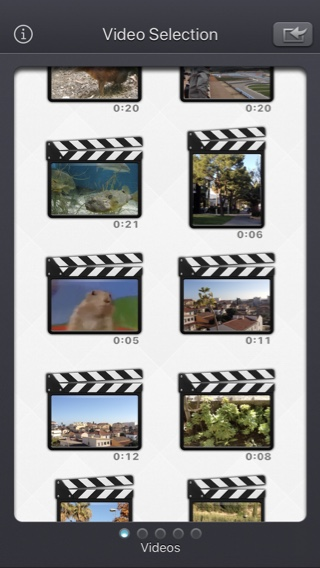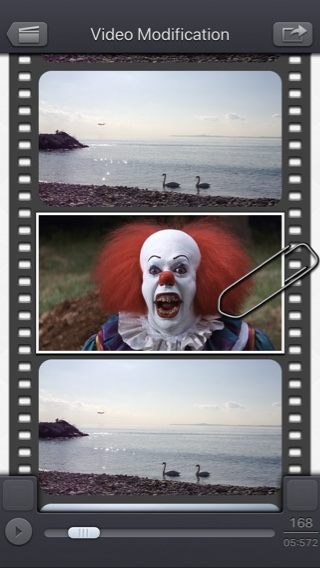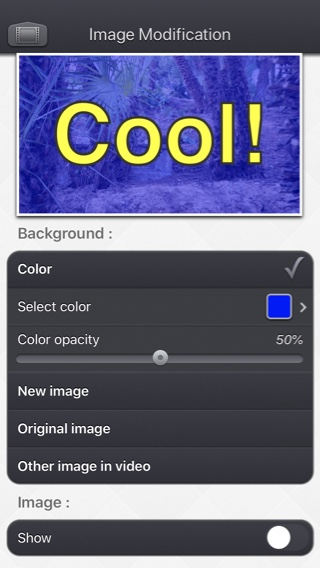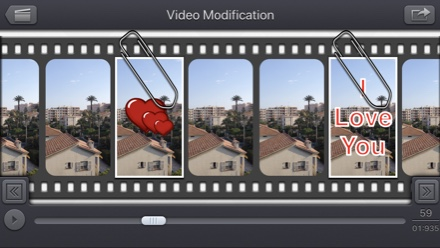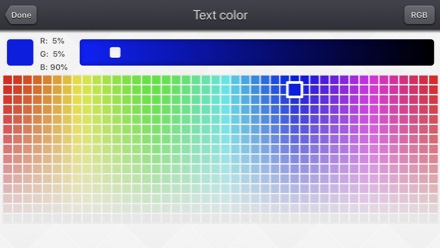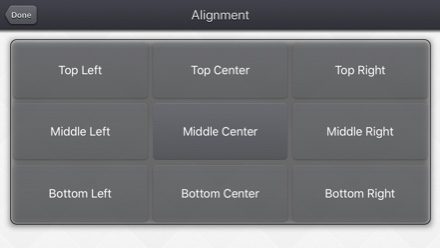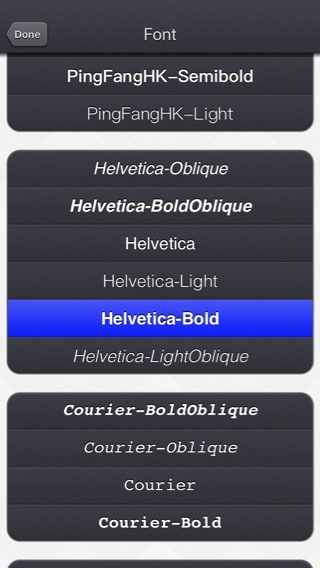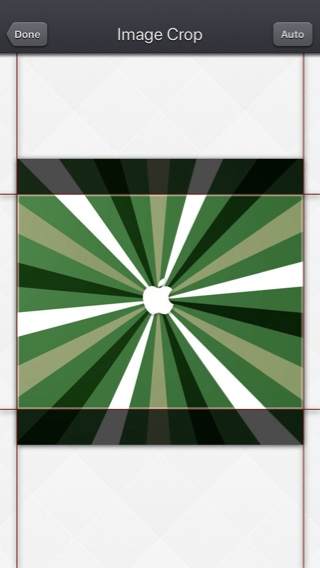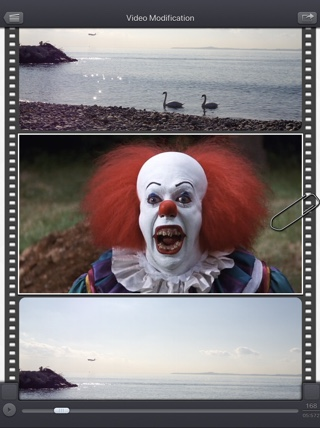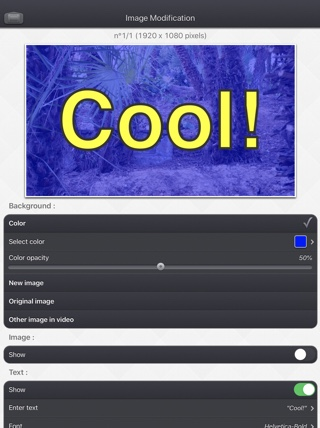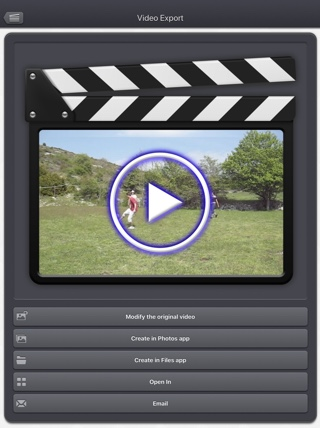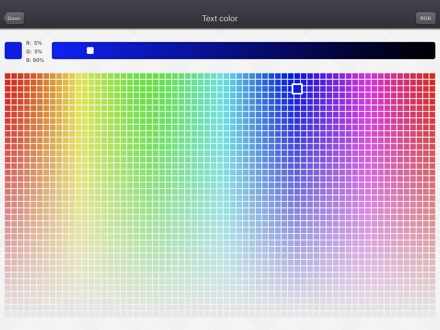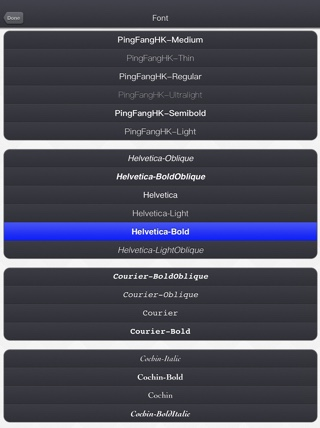# FAQ

• Q : Can you explain how to use the application?
• Q : The app is taking a lot of disk space. Is there a cache cleaning function?
• Q : The videos in my Camera Roll are not accessible. How to solve that problem?
• Q : The videos imported from my computer are not accessible. How to solve that problem?
• Q : How can I delete videos that have been loaded?
• Q : What video formats can be read?

# History

## v5.2.2

• Small fixes and improvements.

# Availability

Subliminal Video can be purchased on the App Store